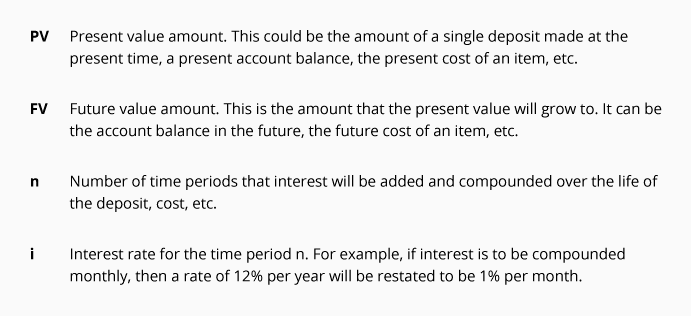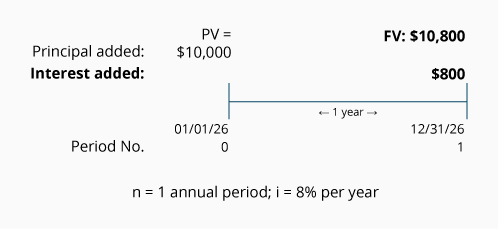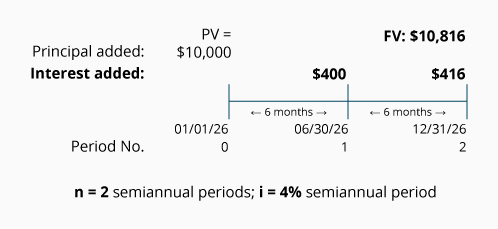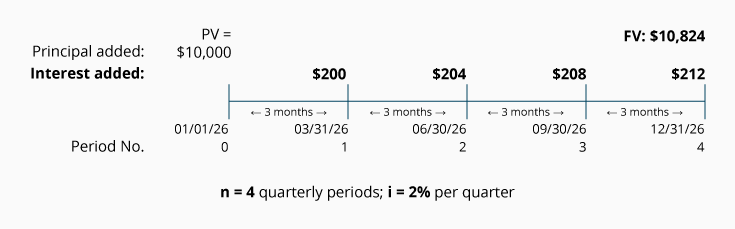## Introduction to Future Value of a Single Amount (FV)

If left undisturbed, a single amount deposited today into your savings account will grow to a larger balance. That future balance is referred to as a future value or FV. Over a long period of time, the future value of that single deposit can grow to be a significant amount for two reasons:

1. the initial deposit earns interest, and

Earning interest on the previously earned interest is known as compound interest.

The calculation of future value determines just how much a single deposit, investment, or balance will grow to, assuming it is left untouched and earns compound interest at a specified interest rate. The calculation of the future value of a single amount can also be used to predict what a present cost of an item will grow to at a future date, when the item's cost increases at a constant rate. Additionally, the formula for computing the future value can be used to determine either the interest rate or the length of time necessary to reach a desired future value.

Our explanation of future value will use timelines for each of the many illustrations in order for you to develop a thorough understanding of the future value of a single amount. Throughout our explanation we will utilize future value tables and future value factors. After mastering these calculations of the future value of a single amount, you are encouraged to use a financial calculator or computer software in order to obtain more precision.

The future value of a single amount is mathematically related to the Present Value of a Single Amount, another topic on this website.

## What's Involved in Future Value (FV) Calculations

The future value of a single amount involves four variables:If you know any three of these four variables, you will be able to calculate the unknown amount.

## Visualizing Compound Interest

To illustrate the compounding of interest in the calculation of a future value, we will assume that a single amount of \$10,000 will be deposited into an account on January 1, and it will remain on deposit for one year. The depositor may select one of three accounts and each of the accounts pays interest of 8% per year. However, the three accounts will differ in the compounding of interest as noted here:

• Account #1. The interest will be added to the account at the end of the year. (Interest is compounded annually on December 31.)

• Account #2. The interest will be added to the account at the end of each six-month period. (Interest is compounded semiannually on June 30 and December 31.)

• Account #3. The interest will be added to the account at the end of each calendar quarter. (Interest is compounded quarterly on March 31, June 30, September 30, and December 31.)

The following timelines will allow us to visualize the compounding of interest and its effect on each account's ending balance.

### Account #1.

A single amount of \$10,000 is deposited on January 1, 2023 and will remain in the account until December 31, 2023. The account will earn interest of 8% per year compounded annually. The timeline showing this information appears here:The timeline shows the single deposit of \$10,000 as the present value and occurring at time period 0. (Time period 0 is the present time or it is the beginning of the first time period.) The timeline also shows that the interest earned during the year 2023 is \$800 (\$10,000 x 8%) and it is added on December 31, 2023. The result is a future value at December 31, 2023 of \$10,800.

### Account #2.

A single amount of \$10,000 is deposited on January 1, 2023 and will remain in the account until December 31, 2023. The account will earn interest at 8% per year but the interest is compounded semiannually. Because interest will be compounded semiannually, the variables n and i must be stated in six-month or semiannual terms as shown in the following timeline:Again, the \$10,000 is the present value shown at time period 0. The timeline also shows that \$400 (\$10,000 x 4%; or \$10,000 x 8% x 0.5 year) of interest is added to the account on June 30, 2023. After the interest is added to the account, the new balance of \$10,400 will earn interest during the second half of the year—resulting in interest of \$416 (\$10,400 x 4% = \$416) added on December 31, 2023. The result is a future value at December 31, 2023 of \$10,816.

### Account #3.

A single amount of \$10,000 is deposited on January 1, 2023 and will remain in the account until December 31, 2023. The account will earn interest at 8% per year but the interest is compounded quarterly. As a result the variables n and i must be thought of in terms of quarters or three-month periods as shown in the following timeline:The present value of \$10,000 will be earning compounded interest every three months. During the first quarter, the account will earn \$200 (\$10,000 x 2%; or \$10,000 x 8% x 3/12 of a year.) and will result in a balance of \$10,200 on March 31. During the second quarter of 2023 the account will earn interest of \$204 based on the account balance as of March 31, 2023 (\$10,200 x 2% per quarter). The interest for the third quarter is \$208 (\$10,404 x 2%) and the interest for the fourth quarter is \$212 (\$10,612 x 2%). The result is a future value of \$10,824 at December 31, 2023.